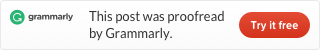Categories

# LeetCode 350. Intersection of Two Arrays II

Given two arrays, write a function to compute their intersection.

Example 1:

```Input: nums1 = [1,2,2,1], nums2 = [2,2]
Output: [2,2]
```

Example 2:

```Input: nums1 = [4,9,5], nums2 = [9,4,9,8,4]
Output: [4,9]```

Note:

• Each element in the result should appear as many times as it shows in both arrays.
• The result can be in any order.

This problem is related to 349. intersection of Two Arrays, but it is more complex.

First, we use two hashmaps to separately count the elements in these two arrays. Then we but two keysets from two hashmaps together into a new keyset.

Then we traverse this new keyset, find the minimum value of the two hashmaps with the same key, it a key don’t exist in one hashmap, it means its value is 0. Then use the minimum to add corresponding amount numbers.

To see more hashtable related problems, see
Problems and Solutions of Hashmap.This site uses Akismet to reduce spam. Learn how your comment data is processed.